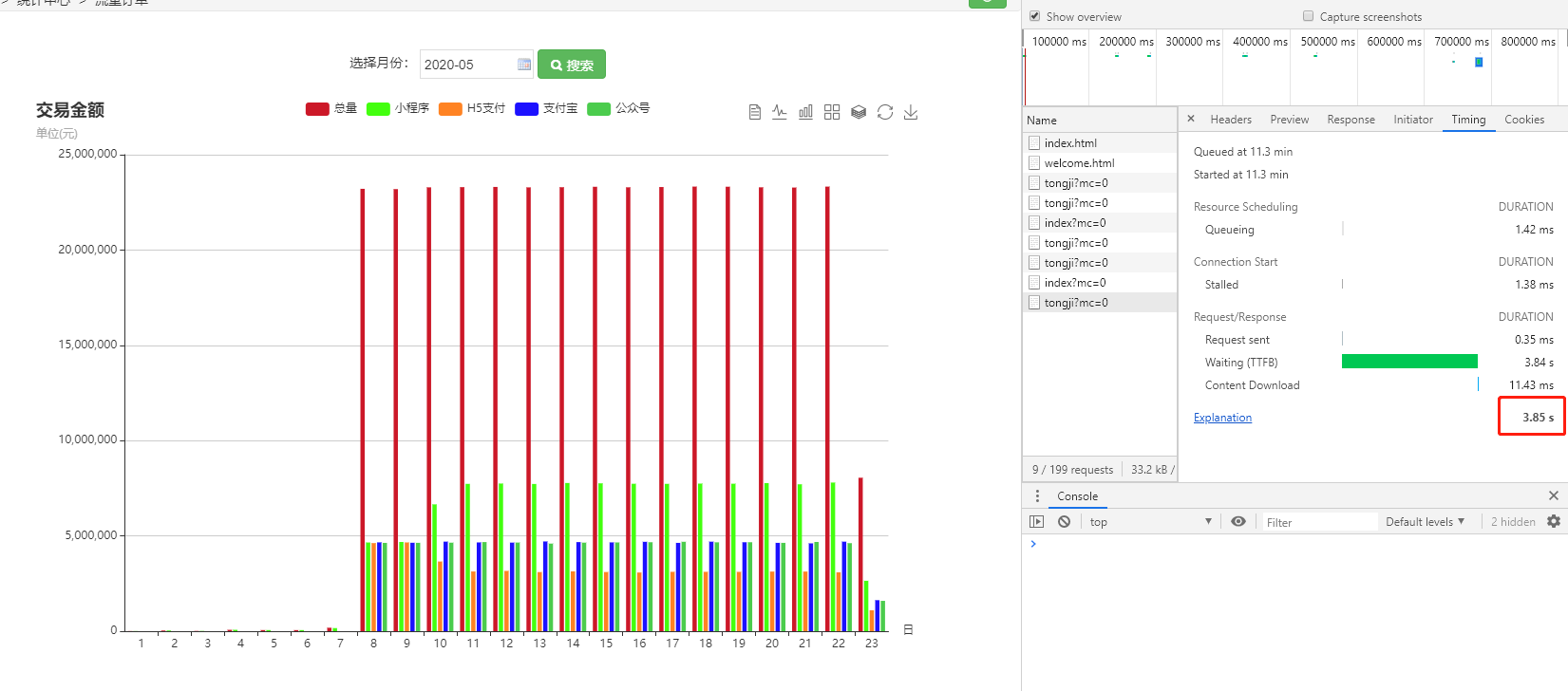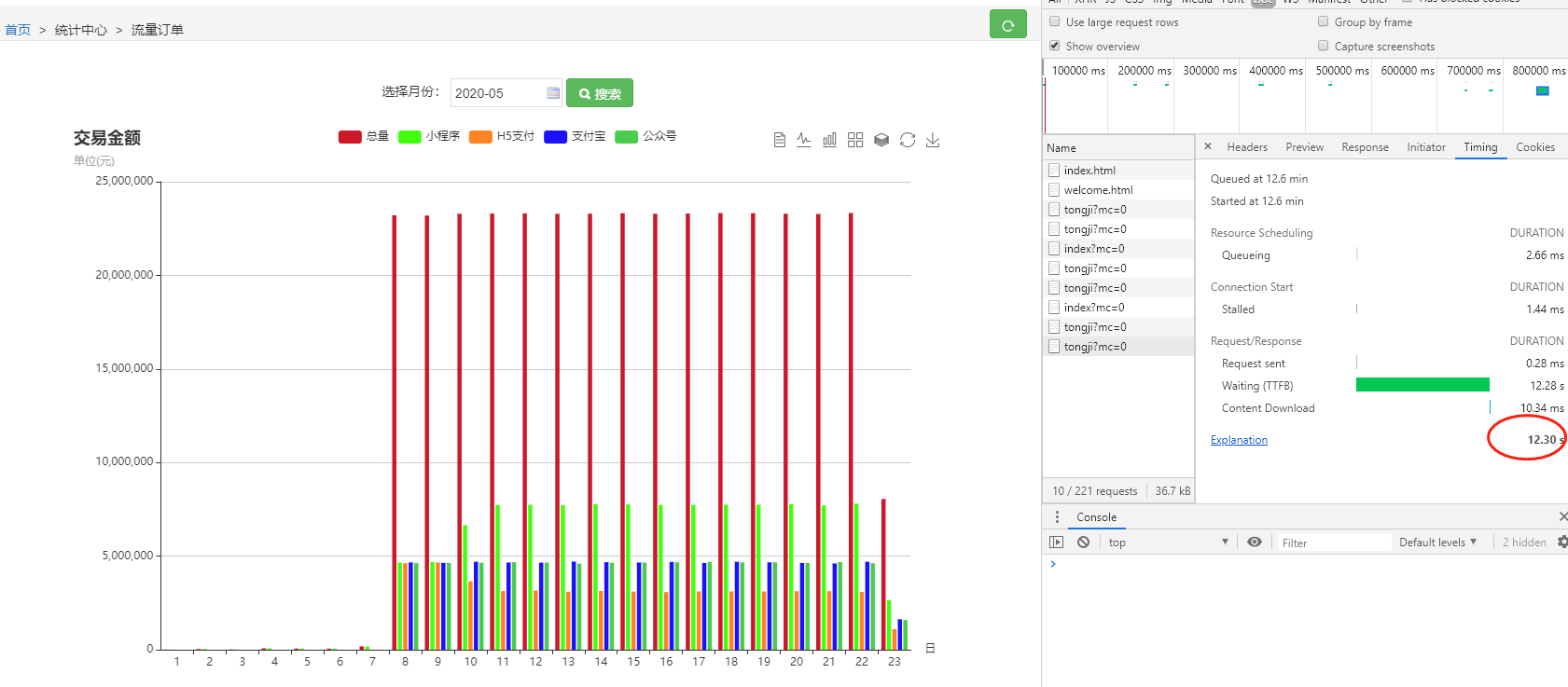# sql查询之统计数据的优化，用IF或者group by 代替多次查询全表

```SELECT SUM(payPrice - refundMoney) total, DATE(payTime) payTime FROM tabl_name
WHERE payTime >= '" . \$stime . "'
AND payTime <= '" . \$etime . "' " . \$where . "
GROUP BY DATE(payTime)
ORDER BY DATE(payTime) ASC```

```SELECT  SUM(IF(payChannel='wx_pub',payPrice - refundMoney,0)) wx_pub,
SUM(IF(payChannel='wx_lite',payPrice - refundMoney,0)) wx_lite,
SUM(IF(payChannel='wx_h5',payPrice - refundMoney,0)) wx_h5,
SUM(IF(payChannel='ali_wap',payPrice - refundMoney,0)) ali_wap,
SUM(payPrice - refundMoney) total,
DATE(payTime) payTime
FROM
table_name
WHERE
payTime >= '" . \$stime . "'
AND payTime <= '" . \$etime . "'

GROUP BY
DATE(payTime)
ORDER BY
DATE(payTime) ASC```